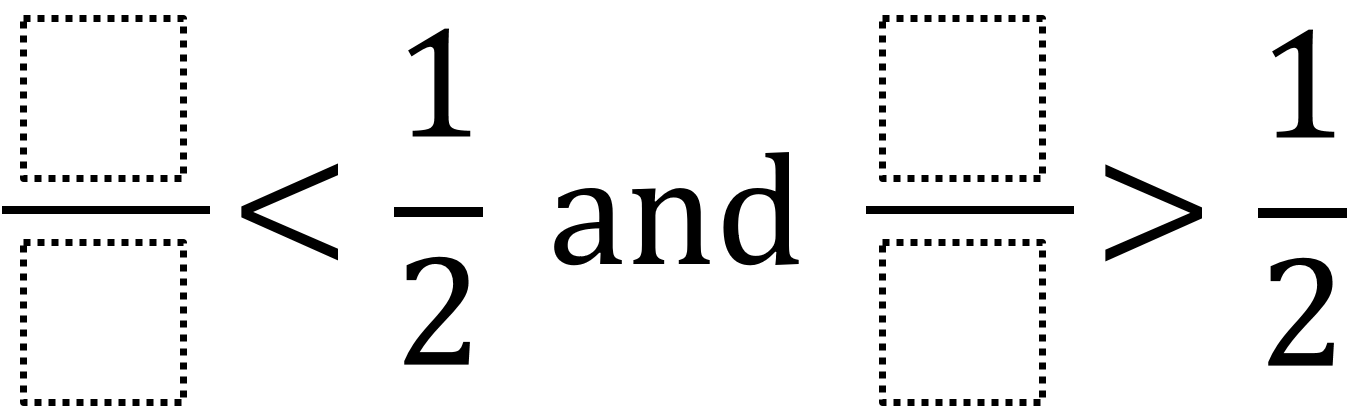Home > Grade 4 > Comparing Fractions

# Comparing Fractions

Directions: Use the digits 1 to 9, at most one time each, to fill in the boxes to create two different fractions: one that is less than one half and one that is more than one half.### Hint

How can we tell when a fraction is less than one half?
How can we find a fraction that is less than one half but has a numerator larger than 1?
How can we tell when a fraction is more than one half?

### Answer

There are many possible answers including

1/4 and 8/9

Other answers you may not expect would be improper fractions that are greater than 1/2 such as 5/2.

Source: Robert Kaplinsky

## Multiplying Differences

Directions: Using the digits 1 to 9 at most one time each, fill in the …

### 4 comments

1.the answer for the first one is 3 over 8 and the answer for the second one is 5 over 8

2.1. 1/3
2. 12/13

3.3/8=5/8 is answer

4.Can you guys add a smaller than 1/8 thing